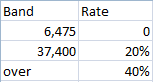## Calculating UK TaxWith this as a table, the following function call can be made from Excel

=IncomeTax(50000.0,TaxRates)

To calculate the tax paid. TaxRates is the range above.

The code for this is as follows.

`Function IncomeTax(income As Double, rates As Range) As Double    Dim i As Integer    Dim tax As Double    tax = 0    For i = 1 To rates.Rows.Count - 1        tax = tax + Application.Min(income, rates.Cells(i, 1)) * rates.Cells(i, 2)        income = Application.Max(0, income - rates.Cells(i, 1))    Next i    IncomeTax = tax + income * rates.Cells(rates.Rows.Count, 2)End Function`